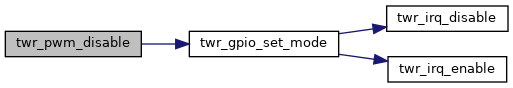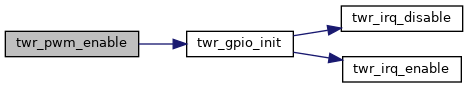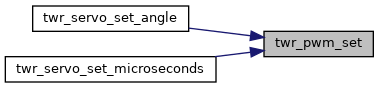Firmware SDK
twr_pwm

Driver for PWM generation. More...

## Enumerations

enum  twr_pwm_channel_t {
TWR_PWM_P0 = TWR_GPIO_P0,
TWR_PWM_P1 = TWR_GPIO_P1,
TWR_PWM_P2 = TWR_GPIO_P2,
TWR_PWM_P3 = TWR_GPIO_P3,
TWR_PWM_P6 = TWR_GPIO_P6,
TWR_PWM_P7 = TWR_GPIO_P7,
TWR_PWM_P8 = TWR_GPIO_P8,
TWR_PWM_P12 = TWR_GPIO_P12,
TWR_PWM_P14 = TWR_GPIO_P14
}
PWM channels.

enum  twr_pwm_tim_t {
TWR_PWM_TIM2_P0_P1_P2_P3,
TWR_PWM_TIM3_P6_P7_P8,
TWR_PWM_TIM21_P12_P14
}
PWM timers. More...

## Functions

void twr_pwm_init (twr_pwm_channel_t channel)
Initialize PWM timers based on GPIO pin. More...

void twr_pwm_enable (twr_pwm_channel_t channel)
Enable PWM output on GPIO pin. More...

void twr_pwm_disable (twr_pwm_channel_t channel)
Disable PWM output on GPIO pin. More...

void twr_pwm_set (twr_pwm_channel_t channel, uint16_t pwm_value)
Set PWM value for GPIO pin. More...

void twr_pwm_tim_configure (twr_pwm_tim_t tim, uint32_t resolution_us, uint32_t period_cycles)
Reconfigure TIM3. More...

## Detailed Description

Driver for PWM generation.

## ◆ twr_pwm_tim_t

 enum twr_pwm_tim_t

PWM timers.

Each timer controls a group of output PWM channels. The change of the specific timer affects also the other related PWM channels.

Definition at line 33 of file twr_pwm.h.

## ◆ twr_pwm_disable()

 void twr_pwm_disable ( twr_pwm_channel_t channel )

Disable PWM output on GPIO pin.

Parameters

Definition at line 188 of file twr_pwm.c.

Here is the call graph for this function:## ◆ twr_pwm_enable()

 void twr_pwm_enable ( twr_pwm_channel_t channel )

Enable PWM output on GPIO pin.

Parameters

Definition at line 174 of file twr_pwm.c.

Here is the call graph for this function:## ◆ twr_pwm_init()

 void twr_pwm_init ( twr_pwm_channel_t channel )

Initialize PWM timers based on GPIO pin.

Parameters
 [in] channel GPIO channel

Definition at line 141 of file twr_pwm.c.

## ◆ twr_pwm_set()

 void twr_pwm_set ( twr_pwm_channel_t channel, uint16_t pwm_value )

Set PWM value for GPIO pin.

Parameters

Definition at line 193 of file twr_pwm.c.

Referenced by twr_servo_set_angle(), and twr_servo_set_microseconds().

Here is the caller graph for this function:## ◆ twr_pwm_tim_configure()

 void twr_pwm_tim_configure ( twr_pwm_tim_t tim, uint32_t resolution_us, uint32_t period_cycles )

Reconfigure TIM3.

Parameters

Definition at line 115 of file twr_pwm.c.